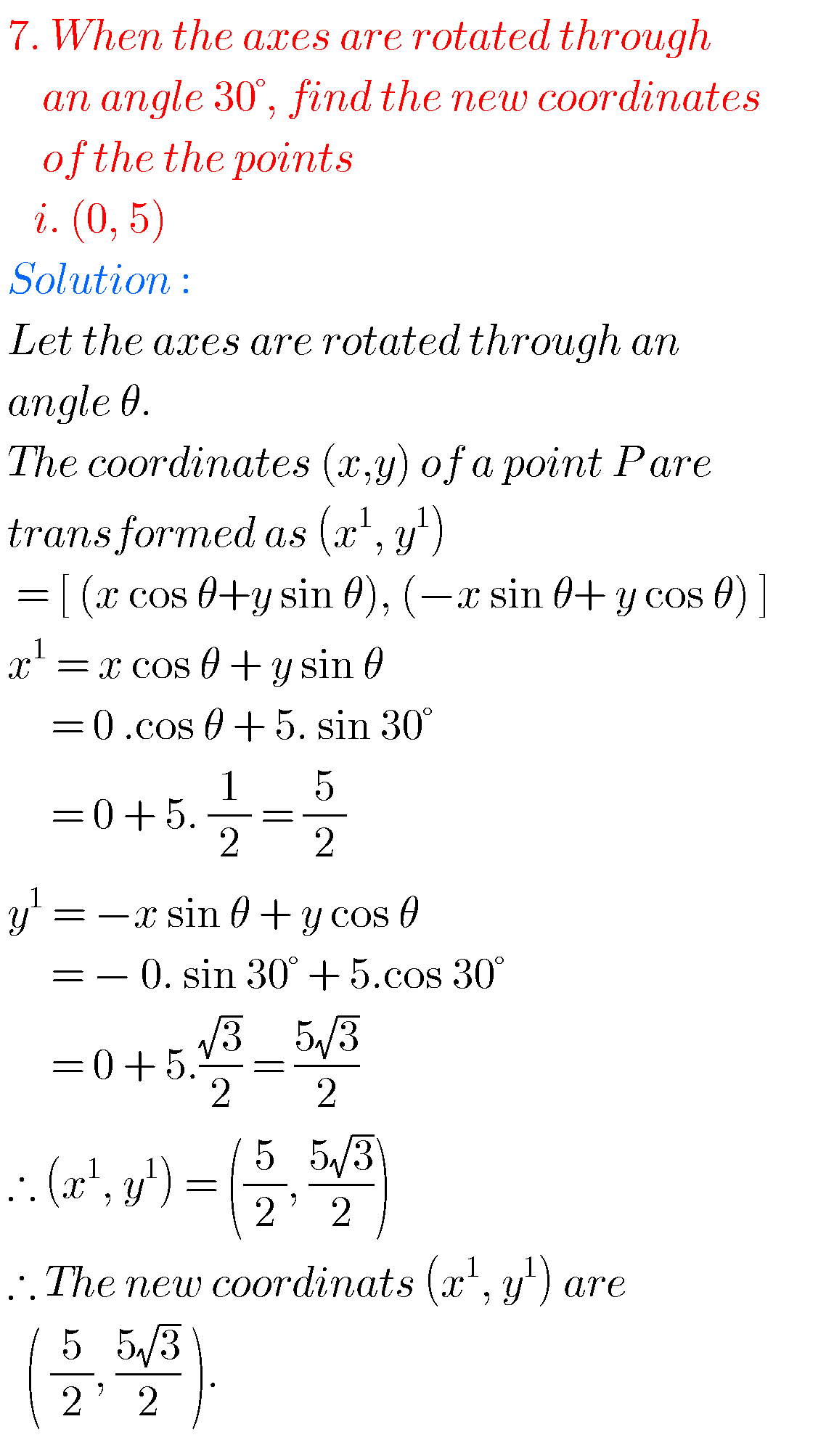This author has not yet filled in any details.
So far Math Tutor has created 57 blog entries.

## Inter maths solutions for Theory of Equations,exercise 4(a),4(b),4(c),4(d) chapter 4 intermediate maths IIA

Inter maths solutions for Theory of Equations Intermediate mathematics IIA chapter 4 theory of equations exercises 4(a), 4(b), 4(c) and 4(d) (text book) solutions are given. These solutions are very easy to understand. First study the text book lessons very well. Then observe solutions and try them in your own method. You can also see [...]

## Inter maths solutions for IIA random variables and probability distributions

Inter maths solutions for IIA random variables and probability distributions Intermediate mathematics IIA chapter 10 random variables and probability distrbutions exercises 10(a) and 10(b) solutions are given. These solutions are very easy to understand. First study the text book lessons very well. Then observe solutions and try them in your own method. You can also [...]

## Inter maths solutions for quadratic expressions,exercise 3(b) chapter 3 second year inter maths IIA

Inter maths solurions for quadratic expressions Intermediate second year mathematics IIA quadratic equations text book exercise 3(b) solutions are given. These solutions are very easy to understand. Study the text book. Observe the solutions and try them in your own methods  You can see the solutions for maths IIA inter  1. Complex numbers 2. De Mouvre's theorem [...]

By | 2019-10-16T15:53:16+05:30 October 16th, 2019|Inter mathematics, mathematics, solutions for inter mathematics|0 Comments
•Inter maths 1b solutions
Gallery

## Inter maths 1b solutions

Inter maths 1b solutions Inter maths solutions for 1b for examination purpose are given. These solutions are very easy to understand.  Study the text book number of times. Observe the solutions and try them in your own methods. Intermediate mathematics 1b solutions Coordinate geometry and calculus solutions inter 1. Locus 2. Transformation of axis 3. The straight line [...]

## Inter maths IIA solutions for quadratic expressions exercise 3(c),chapter 3 intermediate 2nd year 2a

Inter maths IIA solutions for quadratic expressions exercise 3(c) Intermediate second year mathematics quadratic expressions exercise 3(c) solutions ( for text book problems) are given below. These solutions are very easy to understand. You can also see the solurions for first year maths 1A and 1B for examination purpose. Inter maths 1A solutions   1. Functions 2. Mathematical [...]

By | 2019-10-16T15:51:22+05:30 October 16th, 2019|Inter mathematics, mathematics, solutions for inter mathematics|0 Comments

## Inter maths solution for complex numbers,intermediate 2nd year maths 2a chapter 1 solutions

Inter maths solutions for IIA complex numbers Intermediate 2nd year maths chapter 1 solutions for some problems. These solutions are very easy to understand. You can see the solutions for inter 1a 1. Functions 2. Mathematical induction 3. Matrices 4. Addition of vectors 5. Trigonometric ratios upto transformations 1 6. Trigonometric ratios upto transformations 2 7. Trigonometric equations 8. Inverse trigonometric functions 9. Hyperbolic [...]

By | 2019-10-16T15:50:41+05:30 October 16th, 2019|Inter mathematics, mathematics, solutions for inter mathematics|0 Comments

## Inter maths solution for De Moivre’s theorem,intermediate 2nd year mathematics IIA(2a) chapter 2 solutions

Inter maths solution for De Moivre's theorem Mathematics intermediate second year maths IIA solutions for some problems. These are very easy to understand. Inter maths solutions for first year 1a and 1b for examination purpose. Inter maths 2a solutions for complex numbers and de moivre's theorem. Inter first year 1a and 1b solutions are also [...]

By | 2019-10-16T15:48:30+05:30 October 16th, 2019|Inter mathematics, mathematics, solutions for inter mathematics|0 Comments

## Inter maths solutions for Quadratic expressions second year,intermediate maths 2a(IIA) chapter 3 exercise 3(a) text book solutions

Inter maths solutions for Quadratic expressions second year Intermediate mathematics second year solutions for quadratic expressions for some problems. These solutions are very easy to understand. These are helpful for examination purpose.  You can see the solutions for maths IIA inter  1. Complex numbers 2. De Mouvre's theorem 3. Quadratic expressions exercise 3(a) Exercise 3(b) Exercise 3(c) 4. Partial [...]

By | 2019-10-16T15:47:47+05:30 October 16th, 2019|Inter mathematics, mathematics, solutions for inter mathematics|0 Comments

## 1st inter exercise 6(a) solutions maths 1A ,chapter 6 trigonometric ratios upto transformations

1st inter maths chapter 6 ecercise 6(a) solutions Intermediate mathematics 1st year chapter 6 trigonometric ratios upto transformations textbook solutions for exercise 6(a). These solutions are very easy to understant. Exercise 1(b) inter maths 1A You can also see the solutions for inter maths for examination purpose. Inter first year 1a and 1b solutions are [...]

## Inter maths solutions for exercise 6(b) first year,trigonometric ratios upto transformations solutions for 6(b) IA

Inter maths solution for exercise 6(b) first year Intermediate first year mathematics solutions for trigonometric ratios upto transformations exercise 6(b). The solutions are very easy to understand. You can also see the solutions for inter maths for examination purpose.  Inter first year 1a and 1b solutions are also available here. Inter maths 1A  1. Functions 2. Mathematical [...]